# Problem 52921. Easy Sequences 40: Quadratic Congruence

Quadratic Congruence is a modular equation of the form: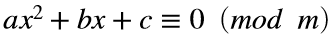.
In this exercise you will be given a vector containing the coefficients of a quadratic polynomial (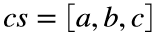), and a modulus base (m). Using these data, create a function that outputs the pair (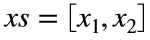), which are the 'primitive' solutions to the quadratic congruence.
For example consider the congruence: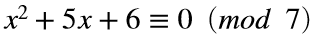, the solution is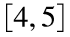, since: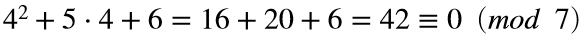, and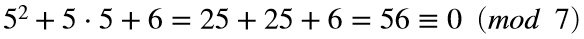.
NOTE: A primitive modulus to base m, can only have values from 0 to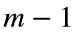. This is a simplified problem, in which the quadratic polynomials given in the test suite, are all factorable, and the modulus base are all odd primes.

### Solution Stats

50.0% Correct | 50.0% Incorrect
Last Solution submitted on Apr 18, 2023

### Community Treasure Hunt

Find the treasures in MATLAB Central and discover how the community can help you!

Start Hunting!